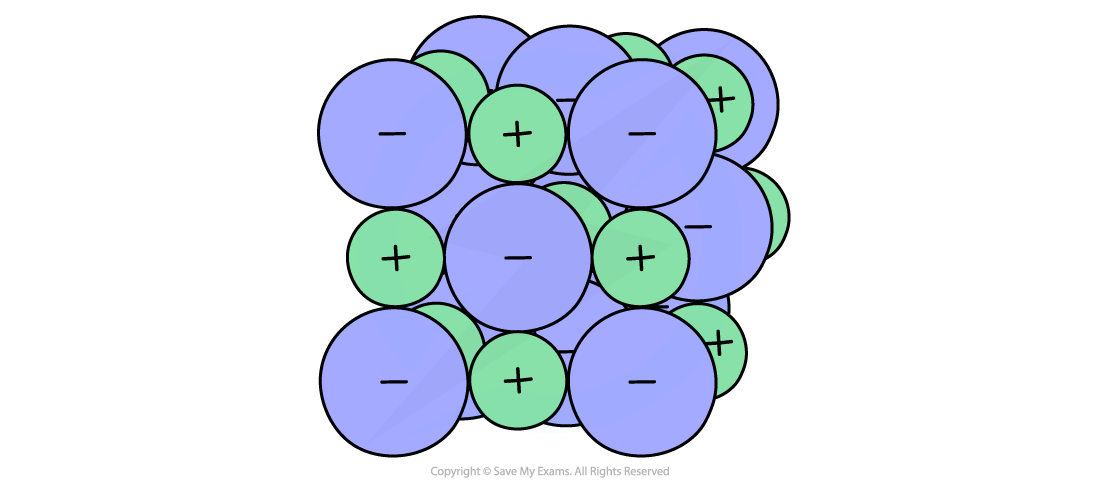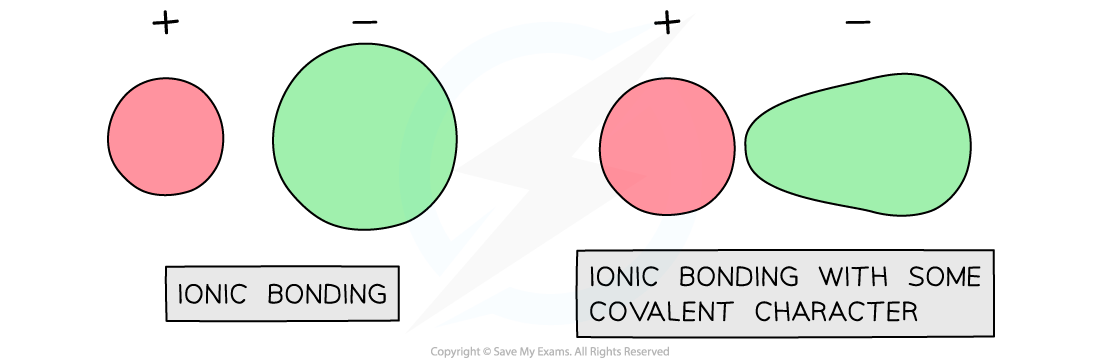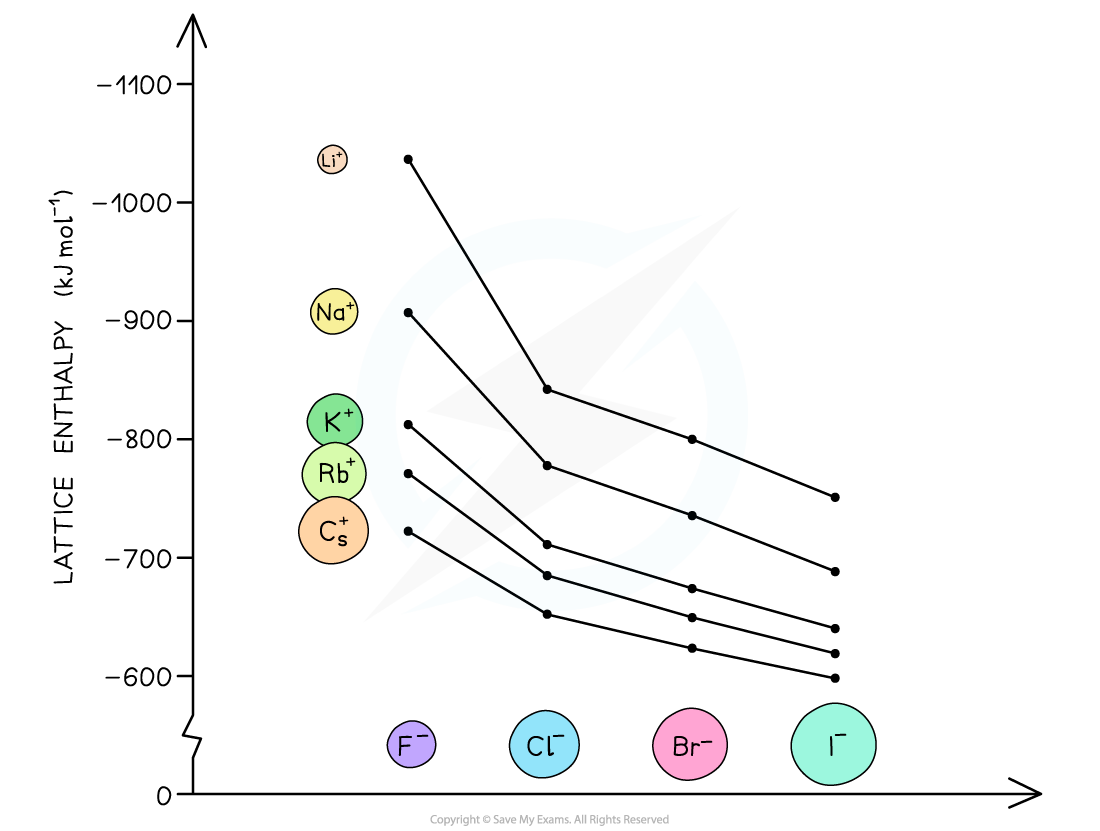# AQA A Level Chemistry复习笔记5.1.4 Comparing Lattice Enthalpies

### Comparing Lattice Enthalpies

#### How accurate are lattice enthalpies?

• It is possible to calculate a theoretical value for the lattice enthalpy of an ionic solid. To do this you need to know
• the geometry of the ionic solid
• the charge on the ions
• the distance between the ions

• This has been calculated for a number of ionic solids and allows a comparison between theoretical lattice enthalpies and experimental lattice enthalpies obtained from Born-Haber cycles

Table comparing theoretical and experimental lattice enthalpies• You can see from the table that there is quite close agreement between the two values for the lattice enthalpy of sodium chloride
• The calculation of the theoretical value is based on an assumption that the substance is a highly ionic compound with only electrostatic attraction between cations and anionsThe ionic model for sodium chloride

• However, the difference between theoretical and experimental lattice enthalpy increases for zinc sulfide
• This suggests that the bonding is not purely ionic and some covalent character is present
• This can be explained as follows:
• Zinc is a smaller ion with a greater charge(+2) than sodium(+1)
• Zinc ions attract electron density towards themselves, distorting the electron cloud and making the bonding slightly covalent
• Sulfide ions are larger ions than chloride ions(-1) with a greater negative charge(-2)
• The electron cloud around sulfide ions is more easily distorted than in chloride ions leading to further covalent characterCovalent character in ionic compounds

• As you move left to right across the period table the lattices become less ionic and more covalent leading to a discrepancy in the lattice enthalpy values
• The result of these analyses provides strong evidence that supports the ionic model for some compounds like sodium chloride

#### Exam Tip

The distortion of the electron clouds is known as polarisation and illustrates that bonding is not either pure ionic or covalent, but rather a continuum between the two extremes.

#### Factors affecting lattice enthalpy

• The two key factors which affect lattice energy, ΔHlattꝋ, are the charge and radius of the ions that make up the crystalline lattice

• The lattice energy becomes less exothermic as the ionic radius of the ions increases
• This is because the charge on the ions is more spread out over the ion when the ions are larger
• The ions are also further apart from each other in the lattice
• The attraction between ions is between the centres of the ions involved, so the bigger the ions the bigger the distance between the centre of the ions

• Therefore, the electrostatic forces of attraction between the oppositely charged ions in the lattice are weaker
• For example, the lattice energy of caesium fluoride (CsF) is less exothermic than the lattice energy of potassium fluoride (KF)
• Since both compounds contain a fluoride (F-) ion, the difference in lattice energy must be due to the caesium (Cs+) ion in CsF and potassium (K+) ion in KF
• Potassium is a Group 1 and Period 4 element
• Caesium is a Group 1 and Period 6 element
• This means that the Cs+ ion is larger than the K+ ion
• There are weaker electrostatic forces of attraction between the Cs+ and F- ions compared to K+ and F- ions
• As a result, the lattice energy of CsF is less exothermic than that of KFThe lattice energies get less exothermic as the ionic radius of the ions increases

#### Ionic charge

• The lattice energy gets more exothermic as the ionic charge of the ions increases
• The greater the ionic charge, the higher the charge density
• This results in stronger electrostatic attraction between the oppositely charged ions in the lattice
• As a result, the lattice energy is more exothermic
• For example, the lattice energy of calcium oxide (CaO) is more exothermic than the lattice energy of potassium chloride (KCl)
• Calcium oxide is an ionic compound which consists of calcium (Ca2+) and oxide (O2-) ions
• Potassium chloride is formed from potassium (K+) and chloride (Cl-) ions
• The ions in calcium oxide have a greater ionic charge than the ions in potassium chloride
• This means that the electrostatic forces of attraction are stronger between the Ca2+ and O2- compared to the forces between K+ and Cl-
• Therefore, the lattice energy of calcium oxide is more exothermic, as more energy is released upon its formation from its gaseous ions
• Ca2+ and O2- are also smaller ions than K+ and Cl-, so this also adds to the value for the lattice energy being more exothermic